>
>
>

# 快速排序为什么这么快？

##### 云起科技：2021-01-25 16:39:58    阅读数：253## 快速排序

• 把小于 pivot 的都挪到 pivot 的左边，
• 把大于 pivot 的都挪到 pivot 的右边。

• pivot 左边
• pivot 右边

### 举个例子：{5, 2, 1, 0, 3}.

• 左边的区间用来放小于 pivot 的元素；
• 右边的区间用来放大于 pivot 的元素；
• 中间是未排序区间。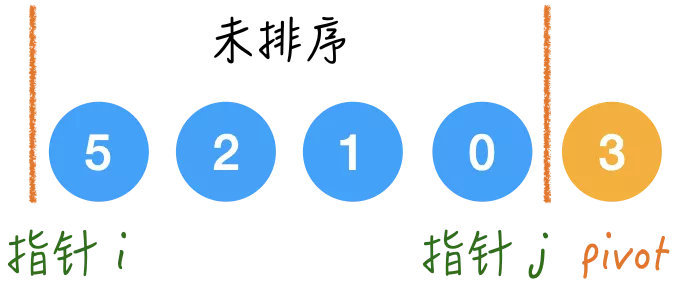• 未排序区间 = [i, j]

• [0, i)：放比 3 小的数；
• (j, array.length -2]：放比 3 大的数

### Step1.

5 > 3, 所以 5 要放在右区间里，所以 5 和 j 指向的 0 交换一下：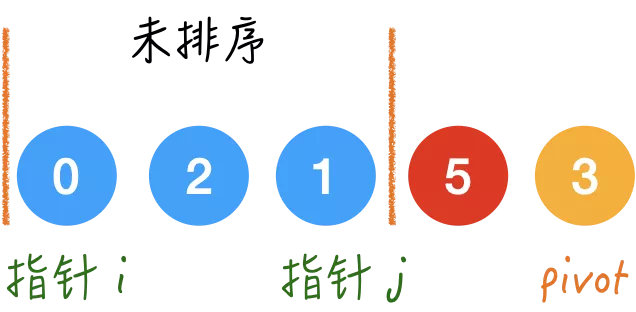### Step2.

0 < 3，所以就应该在左边的区间，直接 i++；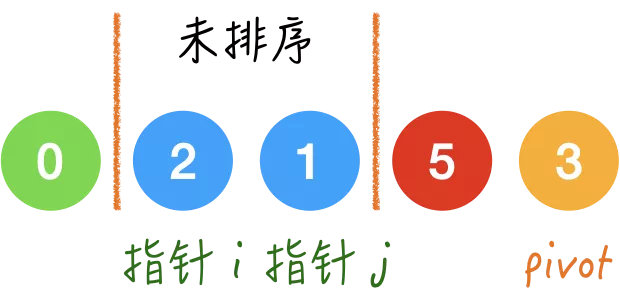### Step3.

2 < 3，同理，i++；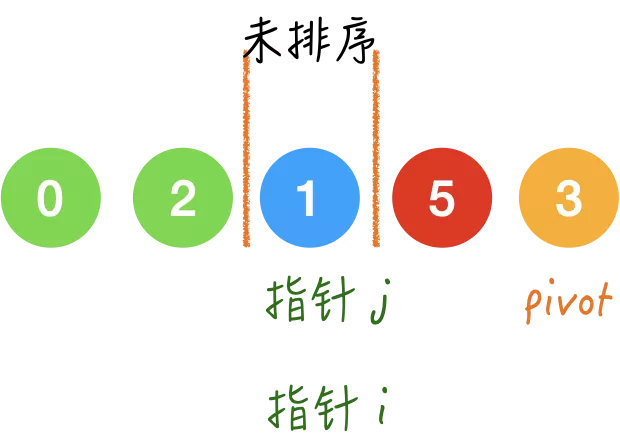### Step4.

1 < 3，同理，i++；1. 回到我们最初选择 pivot的问题，每次都取最后一个，这样做好不好？

1. pivot 放在哪

``````class Solution {
public void quickSort(int[] array) {
if (array == null || array.length <= 1) {
return;
}
quickSort(array, 0, array.length - 1);
}
private void quickSort(int[] array, int left, int right) {
// base case
if (left >= right) {
return;
}

// partition
Random random = new Random(); // java.util 中的随机数生成器
int pivotIndex = left + random.nextInt(right - left + 1);
swap(array, pivotIndex, right);

int i = left;
int j = right-1;
while (i <= j) {
if (array[i] <= array[right]) {
i++;
} else {
swap(array, i, j);
j--;
}
}
swap(array, i, right);

//「分」
quickSort(array, left, i-1);
quickSort(array, i+1, right);
}
private void swap(int[] array, int x, int y) {
int tmp = array[x];
array[x] = array[y];
array[y] = tmp;
}
}

``````

### 1. 均分

#### 时间复杂度

• 每次循环的耗时主要就在这个 while 循环里，也就是 O(right - left)；
• 均分的话那就是 logn 层；
• 所以总的时间是 O(nlogn).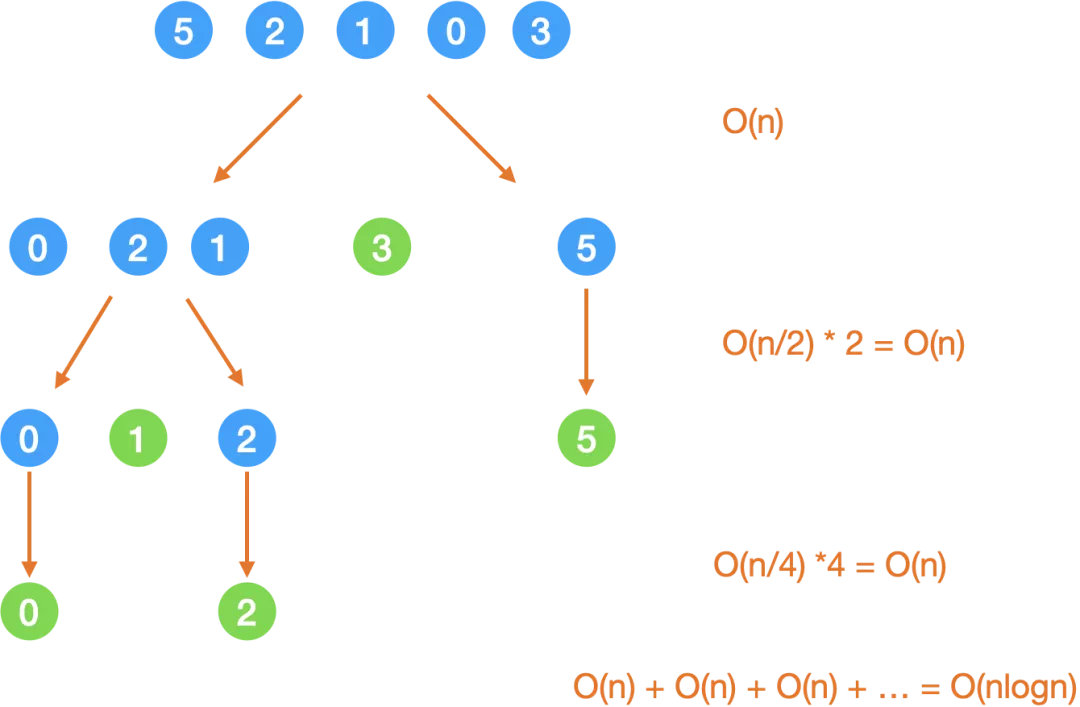#### 空间复杂度

• 递归树的高度是 logn，
• 每层的空间复杂度是 O(1)，
• 所以总共的空间复杂度是 O(logn).

### 2. 最不均匀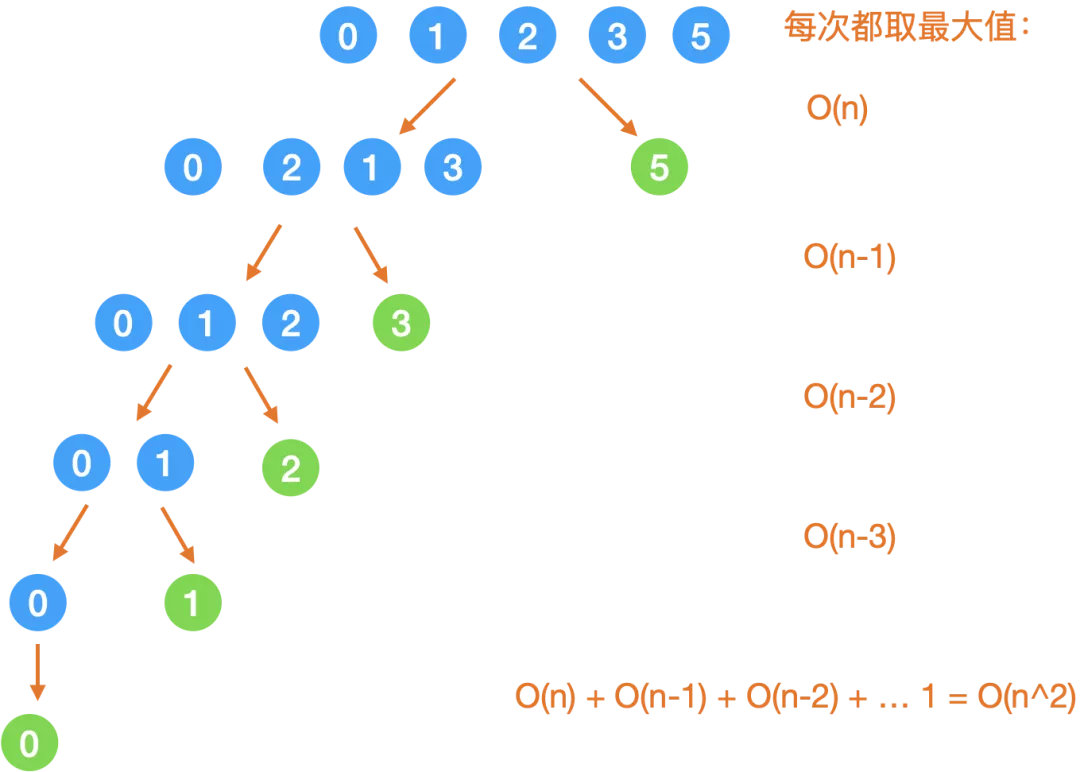### 稳定性

• 为什么对于 primitive type 使用快排

• 因为它速度最快；
• 为什么对于 object 使用归并

• 因为它具有稳定性且快。